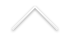Resumo: We will deal with a 1-dimensional reaction-diffusion-convection PDE where the diffusion is of mean-curvature type and both convection and reaction terms are present. We will assume f(0) = 0 = f(1) and search for traveling-wave type solutions u(x; t) = v(x+ct) connecting the two equilibria 0 and 1.

Through the use of a suitable change of variables, we will translate this problem into the search for a solution to a two-point problem in the interval [0; 1], and determine the set of the admissible speeds (i.e., the numbers c for which a solution of this kind exists) for different kinds of reaction and convection terms, thanks to a shooting technique. We will also make some comments about the dependence of such admissible speeds on a viscosity parameter braking the diffusion.

Expositor: Maurizio Garrione
Data: 09/03/2016
Horário: 13:30
Local: Sala C116

Heteroclinic traveling-wave type solutions for reaction-diffusion-convection equations with saturating diffusion